# tightPosition

Position of axes plotting area

## Syntax

``pos = tightPosition(ax)``
``pos = tightPosition(ax,IncludeLabels=true)``

## Description

example

````pos = tightPosition(ax)` returns the position of the rectangle that circumscribes the plotting area of the axes `ax`. The result is a four-element vector of the form ```[left bottom width height]``` that describes the position and size of the rectangle in the units specified in the `Units` property of the axes object. By default, the units are normalized values between `0` and `1`.```

example

````pos = tightPosition(ax,IncludeLabels=true)` returns the position of the rectangle that surrounds the title, subtitle, tick labels, and axis labels. If any of the labels are close to the edge of the figure, the position rectangle might fall outside the bounds of the figure.```

## Examples

collapse all

Plot a spiral. Use the `axis` function to adjust the plot box so that the x- and y-scales are equal and so that the plot box surrounds the spiral tightly.

```t = linspace(0,10,100); ax = axes; plot(t.*sin(t),t.*cos(t)) axis equal tight```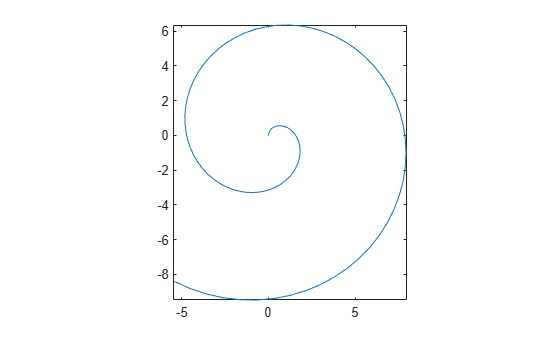Get the position vector, and use that vector to draw a red rectangle that circumscribes the plotting area. For Cartesian axes, the plotting area is the area inside the plot box. This rectangle is coincident with the plot box.

```pos = tightPosition(ax); annotation("rectangle",pos,Color="red",LineWidth=2)```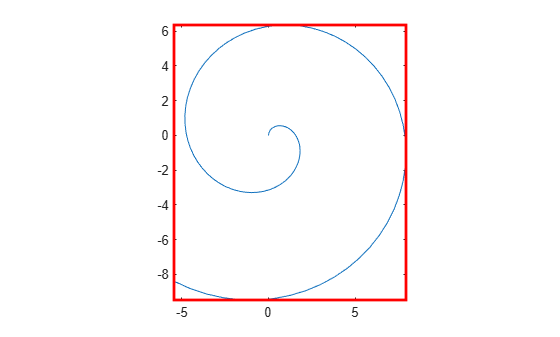Get the position vector that includes the axis tick labels, and use that vector to draw a blue rectangle around the plot box and the tick labels.

```poslab = tightPosition(ax,IncludeLabels=true); annotation("rectangle",poslab,Color="blue",LineWidth=2)```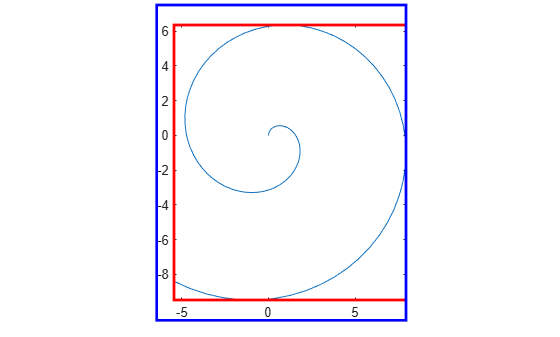Load the `mri` data set and display the MRI image stored in the `D` variable. Use the `axis` function to preserve the aspect ratio of the image.

```figure ax = axes; load mri image(D(:,:,10)) axis image```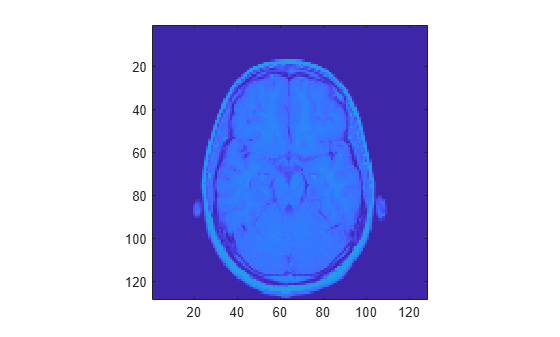Get the position vector, and use that vector to draw a red rectangle that circumscribes the plotting (image) area. For Cartesian axes, the plotting area is the area inside the plot box. This rectangle is coincident with the plot box.

```pos = tightPosition(ax); annotation("rectangle",pos,Color="red",LineWidth=2)```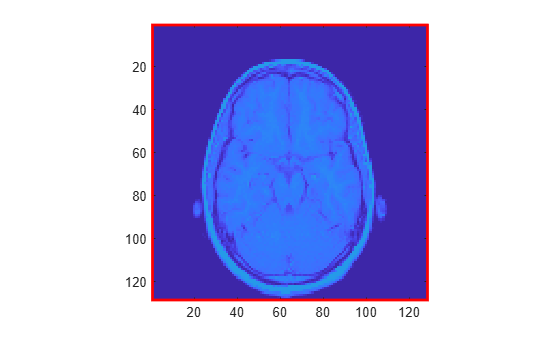Get the position vector that includes the axis tick labels, and use that vector to draw a blue rectangle around the plot box and the tick labels.

```poslab = tightPosition(ax,IncludeLabels=true); annotation("rectangle",poslab,Color="blue",LineWidth=2)```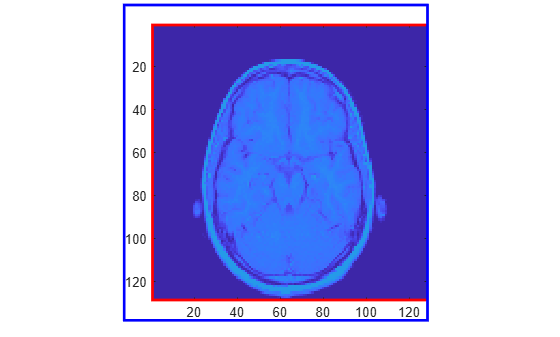## Input Arguments

collapse all

Axes object, specified as an `Axes`, `PolarAxes`, or `GeographicAxes` object.

## Output Arguments

collapse all

Position vector, returned as a four-element vector of the form ```[left bottom width height]```.

This vector specifies the position and size of the circumscribing rectangle in the units specified by the `Units` property of the axes object. By default, the units are normalized values between `0` and `1`.

• The `left` value is the distance between the left edge of the parent container and the left edge of the rectangle. The parent container is typically a figure, panel, or tab.

• The `bottom` value is the distance between the bottom edge of the parent container and the bottom edge of the rectangle.

• The `width` and `height` values define the width and height of the rectangle.

## Version History

Introduced in R2022b